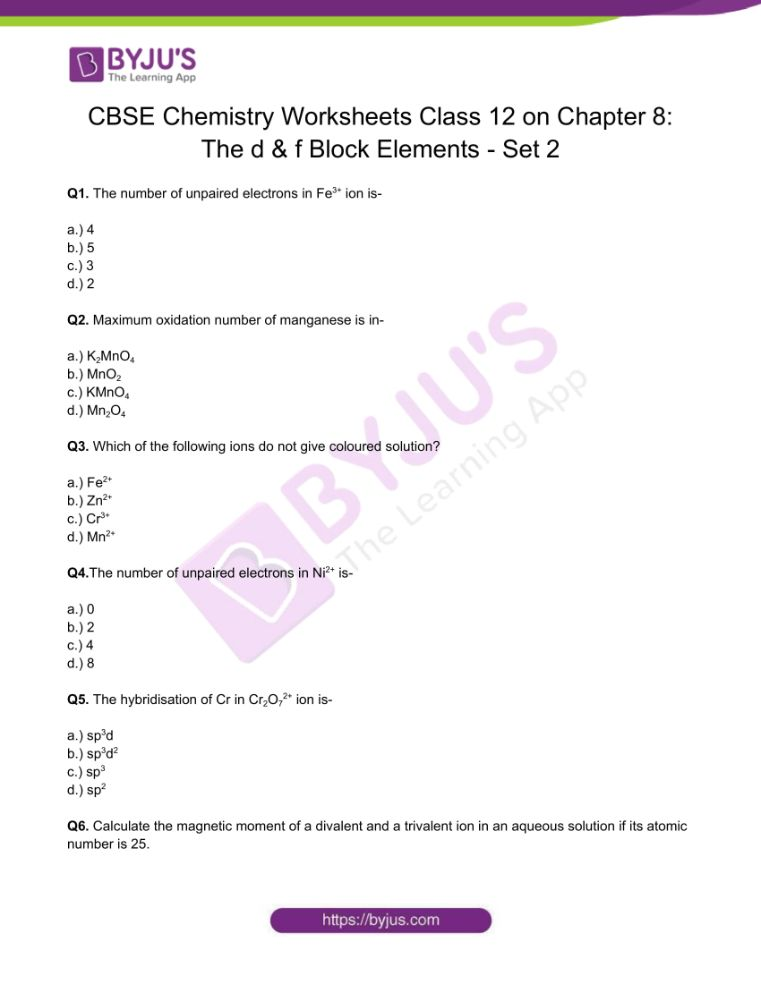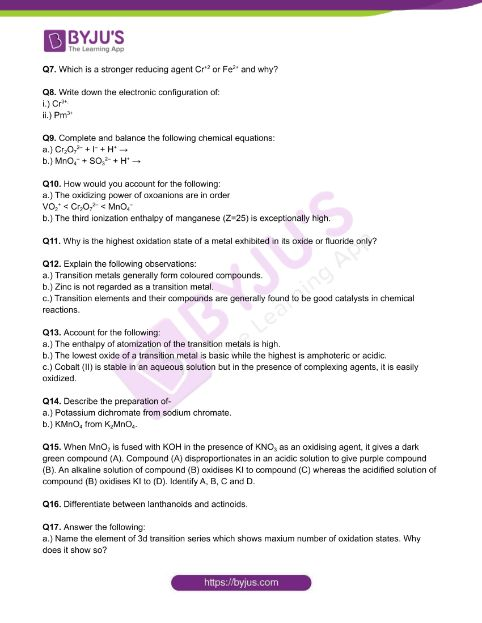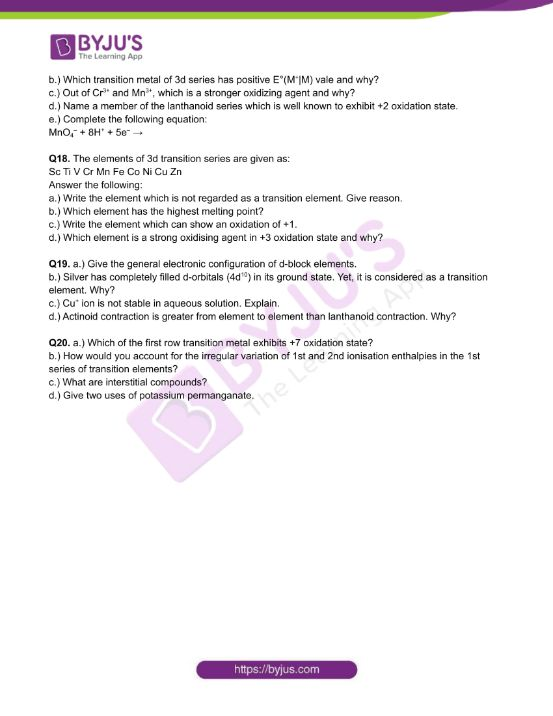Checkout JEE MAINS 2022 Question Paper Analysis : Checkout JEE MAINS 2022 Question Paper Analysis :

# Chemistry Worksheets Class 12 on Chapter 8: The d & f Block Elements with Answers - Set 2

The elements present in the middle of the periodic table from Group 3 to 12 are called d-block elements. The name d- blocks because the last electron enters into the d-orbital of the penultimate shell. These are often called transition elements because their characters lie between highly reactive metallic elements of s-block and nonmetallic elements of p-block elements. There are four series in the d block corresponding to the filling up of 3d, 4d, 5d or 6d orbitals.

• First transition series or 3d series ( Sc, Ti, V, Cr, Mn, Fe, Co, Ni, Cu, Zn)
• Second transition series or 4d series (Y, Zr, Nb, Mo, Tc, Ru, Rh, Pd, Ag, Cd)
• Third transition series or 5d series (La, Hf, Ta, W, Re, Os, Ir, Pt, Au, Hg)
• Fourth transition series or 6d series- incomplete.

On the other hand,f block elements are found outside at the bottom of the periodic table. The name f- blocks because the last electron enters into the f-orbital of the anti-penultimate shell. These are also called inner transition elements. There are 2 series in the f block, corresponding to the filling up of 4f and 5f orbitals.

• Lanthanide series or 4f series (Ce, Pr, Nd, Pm, Sm, Eu, Gd, Tb, Dy, Ho, Er, Tm , Yb)
• Actinide series of 5f series ( Th, Pa, U, Np, Pu, Am, Cm, Bk, Cf, Es, Fm, Md, Mo)

## 👇## CBSE Class 12 Chemistry Chapter 8 The d & f Block Elements Worksheet – Set 2

Q1. The number of unpaired electrons in Fe3+ ion is-

a.) 4

b.) 5

c.) 3

d.) 2

Q2. Maximum oxidation number of manganese is in-

a.) K2MnO4

b.) MnO2

c.) KMnO4

d.) Mn2O4

Q3. Which of the following ions do not give coloured solution?

a.) Fe2+

b.) Zn2+

c.) Cr3+

d.) Mn2+

Q4.The number of unpaired electrons in Ni2+ is-

a.) 0

b.) 2

c.) 4

d.) 8

Q5. The hybridisation of Cr in Cr2O72+ ion is-

a.) sp3d

b.) sp3d2

c.) sp3

d.) sp2

Q6. Calculate the magnetic moment of a divalent and a trivalent ion in an aqueous solution if its atomic number is 25.

Q7. Which is a stronger reducing agent Cr+2 or Fe2+ and why?

Q8. Write down the electronic configuration of:

i.) Cr3+.

ii.) Pm3+

Q9. Complete and balance the following chemical equations:

a.) Cr2O72– + I + H+

b.) MnO4 + SO32– + H+

Q10. How would you account for the following:

a.) The oxidizing power of oxoanions are in order

VO2+ < Cr2O72– < MnO4

b.) The third ionization enthalpy of manganese (Z=25) is exceptionally high.

Q11. Why is the highest oxidation state of a metal exhibited in its oxide or fluoride only?

Q12. Explain the following observations:

a.) Transition metals generally form coloured compounds.

b.) Zinc is not regarded as a transition metal.

c.) Transition elements and their compounds are generally found to be good catalysts in chemical reactions.

Q13. Account for the following:

a.) The enthalpy of atomization of the transition metals is high.

b.) The lowest oxide of a transition metal is basic while the highest is amphoteric or acidic.

c.) Cobalt (II) is stable in an aqueous solution but in the presence of complexing agents, it is easily oxidized.

Q14. Describe the preparation of-

a.) Potassium dichromate from sodium chromate.

b.) KMnO4 from K2MnO4.

Q15. When MnO2 is fused with KOH in the presence of KNO3 as an oxidising agent, it gives a dark green compound (A). Compound (A) disproportionates in an acidic solution to give purple compound (B). An alkaline solution of compound (B) oxidises KI to compound (C) whereas the acidified solution of compound (B) oxidises KI to (D). Identify A, B, C and D.

Q16. Differentiate between lanthanoids and actinoids.

a.) Name the element of 3d transition series which shows maxium number of oxidation states. Why does it show so?

b.) Which transition metal of 3d series has positive E°(M+|M) vale and why?

c.) Out of Cr3+ and Mn3+, which is a stronger oxidizing agent and why?

d.) Name a member of the lanthanoid series which is well known to exhibit +2 oxidation state.

e.) Complete the following equation:

MnO4 + 8H+ + 5e

Q18. The elements of 3d transition series are given as:

Sc Ti V Cr Mn Fe Co Ni Cu Zn

a.) Write the element which is not regarded as a transition element. Give reason.

b.) Which element has the highest melting point?

c.) Write the element which can show an oxidation of +1.

d.) Which element is a strong oxidising agent in +3 oxidation state and why?

Q19. a.) Give the general electronic configuration of d-block elements.

b.) Silver has completely filled d-orbitals (4d10) in its ground state. Yet, it is considered as a transition element. Why?

c.) Cu+ ion is not stable in aqueous solution. Explain.

d.) Actinoid contraction is greater from element to element than lanthanoid contraction. Why?

Q20. a.) Which of the first row transition metal exhibits +7 oxidation state?

b.) How would you account for the irregular variation of 1st and 2nd ionisation enthalpies in the 1st series of transition elements?

c.) What are interstitial compounds?

d.) Give two uses of potassium permanganate.

Download PDF to access answers of Chemistry Worksheet for Class 12 Chemistry Chapter 8: The d & f Block Elements set – 2.